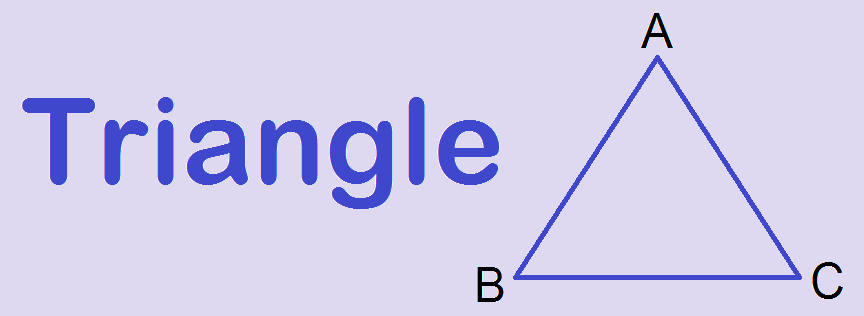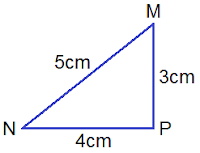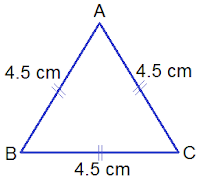# Triangle | What is Triangle?## Triangle | What is Triangle?A closed plane figure formed by three line segments is called a triangle. It is denoted by the symbol Δ

In the figure alongside, PQR is a closed plane figure formed by three line segments PQ, QR and RP. So it is a triangle. It is represented by ΔPQR. The points P, Q and R are called the vertices of the triangle PQR.

### Types of triangles on the basis of sides

On the basis of sides there are three types of triangles:

####i.               Scalene Triangle:

A triangle having non of the sides equal is called a scalene triangle. In the given triangle, MN ≠ NP ≠ PM. So ΔMNP is a scalene triangle.

#### ii.             Isosceles Triangle:

A triangle with having two sides equal is called an isosceles triangle. In the given triangle, PQ = PR = 5 cm. So, ΔPQR is an isosceles triangle.

Note:
Base angles are always equal of an isosceles triangle. In the given figure PQR = PRQ.

####iii.            Equilateral Triangle:

A triangle with having all three sides equal is called an equilateral triangle. In the given triangle, AB = BC = AC = 4.5 cm. So, ΔABC is an equilateral triangle.

Note:
All angles of an equilateral triangle are 60°. An equilateral triangle holds all the properties of an isosceles triangle.

### Types of triangles on the basis of angles

On the basis of angles, there are three types of triangles:

####i.               Acute Angled Triangle:

If all the angles of a triangle are less than 90° or acute angles, then it is called an acute angled triangle. In the given triangle, each angle of the triangle is less than 90°. So, ΔABC is an acute angled triangle.

####ii.             Obtuse Angled Triangle:

The triangle with one angle is obtuse angle or more than 90° is called an obtuse angled triangle. In the given figure, RMN is an obtuse angle. So, ΔMNR is an obtuse angled triangle.

####iii.            Right Angled Triangle:

The triangle with one angle a right angle or equal to 90° is called a right angled triangle.
In the given figure, PQR is 90°. So, ΔPQR is a right angled triangle.

Note:
If a right angled triangle has two sides equal, then it is called a right angled isosceles triangle.

### Properties of triangle:

1.    Sum of three angles of a triangle is always 180°.
2.    Base angles of an isosceles triangle are always equal.
3.    All angles of an equilateral triangle are equal to 60° each.
4.    Base angles of a right angled isosceles triangle are equal to 45° each.
5.    If any two angles of a triangle are equal, then the sides opposite to them are also equal.
6.    The exterior angle of a triangle is equal to the sum of two opposite interior angles.
7.    The sum of two sides of a triangle is greater than the third side.
8.    In any triangle, the side opposite to greater angle is longer than the side opposite to the smaller angle.
9.    The line drawn from the vertex of an isosceles triangle to the mid point of its base is perpendicular to the base.
10.The bisector of the vertical angle of an isosceles triangle is always perpendicular to the base.
11. The line joining mid-points of any two sides of a triangle is parallel to third side and is half the length of third side.

You can comment your questions or problems regarding the triangle here.